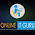### Nan, Infinity and the healing power of DIVIDE

This is a little post about a really simple change that I've found really helpful concerning tables/matrixes showing NaN and Infinity.

What's up with this?

This happens when you have a measure that equates to <a number> / nothing

If your nothing is the number zero then BI shows infinity. More commonly though your nothing is...nothing. BLANK() in terms of DAX (null in SQL). That's when you get NaN which stands for Not a Number.

Note that if your denominator is COUNTROWS and there are no rows in the filter context then DAX returns BLANK not 0.

Solution

The simple solution is to use the DIVIDE function which takes the numerator and denominator as its first two arguments. If the denominator is blank then by default it returns BLANK() (So crucially won't force a row to appear in a table). You can also add a third argument with an alternate result if the numerator can't be divided by the denominator.

So now you don't find / in my measure code because DIVIDE is better.

1.Good Post! Thank you so much for sharing this pretty post

power bi online training
power bi online course